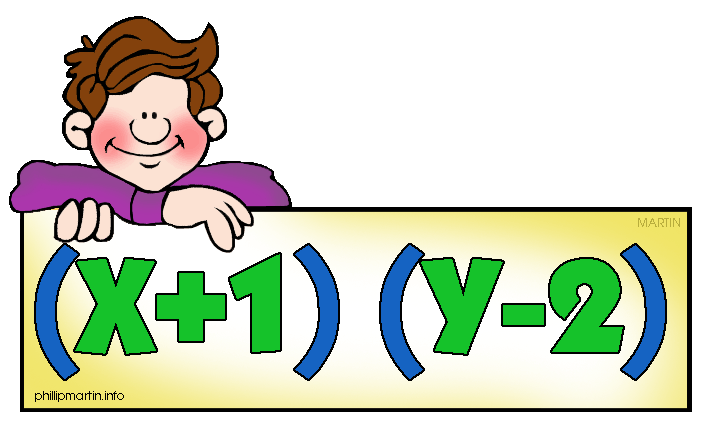#### In this section,

We will be taking a look at the most complex equations. When solving the most complex trig equations, it is important to make sure the equation cannot be simplified. one way you could simplify a trig equation is by factoring and using the zero product property. Take a look:

#### Solving these equations:

• Simplify the equation
• Determine if the equation can be factored
• Use the zero product property

#### Examples:

Ex.1

3 Tan2x + 5Tanx = 0

Tanx (3Tanx + 5) = 0 ←Factor out GCF

Tanx = 0 Tanx = -5/3 ←Set equal to 0

X=0,π Tanx-1=-5/3

Quadrants: II, IV ←Find quadrants
X1=1.03
II = 2.11
IV = 5.25

###### Solutions: {2.11, 5.25}

Ex.2
6sin2x + 7sinx - 5 = 0

(3sinx + 5) (2sinx - 1) = 0 ←Factor

3sinx + 5 = 0 2sinx - 1 = 0 ←Set equal to 0

sinx = -5/3 sinx = ½

Quadrants: I, II ← Find quadrants
XI = π/6
I = π/6
II = 5π/6

#### Practice Problems:

ANSWER KEY
1) Tan2x + 2tanx = -1

2) 2sin2x - 3 sinx + 1 = 0